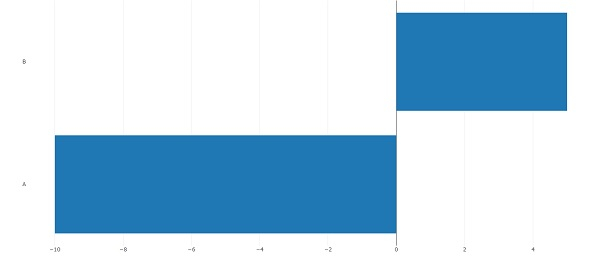# How to create plotly bar chart with negative values in R?

To create plotly bar chart with negative values in R, we can follow the below steps −

• First of all, create a data frame.
• Then, create the bar chart using plotly with horizontal orientation.

## Create the data frame

Let's create a data frame as shown below −

Live Demo

Group<-c("A","B")
Score<-c(-10,5)
df<-data.frame(Group,Score)
df

On executing, the above script generates the below output(this output will vary on your system due to randomization) −

Group Score
1 A   -10
2 B   5

## Create the bar chart with horizontal orientation

Using plot_ly function with Group.orientation for y values set to "h" to create the bar chart −

Group<-c("A","B")
Score<-c(-10,5)
df<-data.frame(Group,Score)
library(plotly)
plot_ly(df,type="bar",x=Score,y=Group,orientation="h")

#### Output